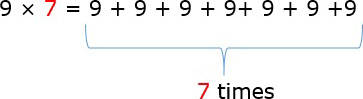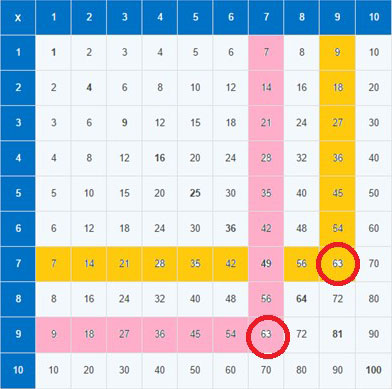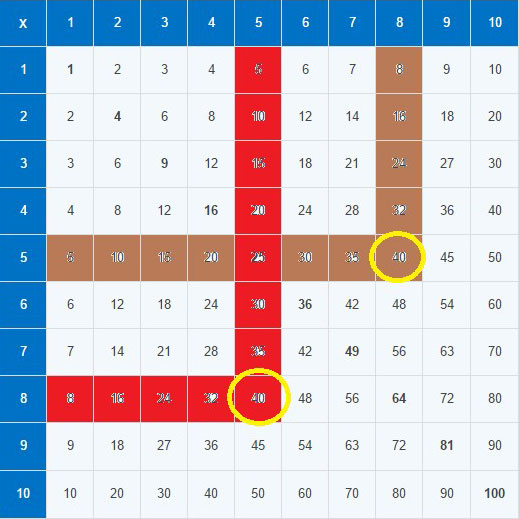# Single Digit Multiplication

When we multiply a single digit by another single digit number, the product is obtained by repeated addition or from memorizing multiplication tables.

For example: In the multiplication 3 × 4, we either repeatedly add 3 four times as 3 + 3 + 3 + 3 = 12 or use multiplication table for 3 to get the answer for 3 × 4 = 12.

Multiply 9 × 7

### Solution

Step 1:

To multiply 9 by 7 means, to add 9 seven times as shown below.Step 2:

But there is no need to do this repeated addition every time. We need to memorize the multiplication tables and write down the product from memory or from the multiplication table as shown below.9 times 7 can be read from the table by looking up the 9th row and 7th column and where they meet is the product 63 (pink color)

7 times 9 can also be read from the table by looking up the 7th row and 9th column and where they meet is the product 63 (dark yellow color)

9 × 7 = 63 and 7 × 9 = 63

Multiply 8 × 5

### Solution

Step 1:

To multiply 8 by 5 means, to add 8 five times as shown below.

8 + 8 + 8 + 8 + 8 = 40

Step 2:In a similar way, 8 × 5 can be looked up in the multiplication table as shown.

8 × 5 = 40 and 5 × 8 = 40